UPSC  >  Permutation, Combination, & Probability- Solved Questions(1995-2020)- 2

# Permutation, Combination, & Probability- Solved Questions(1995-2020)- 2 - Notes | Study UPSC Topic Wise Previous Year Questions - UPSC

 1 Crore+ students have signed up on EduRev. Have you?

Question 1: In how many different ways can all of 5 identical balls be placed in the cells shown below such that each row contains at least 1 ball?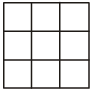(a) 64
(b) 81
(c) 84
(d) 108

There can be two cases :
Case I : When 1 row contains 3 balls and rest two contains 1 ball each.
Now, the row which contains 3 balls can be selected out of the 3 rows in 3C1 ways and in this row number of ways of arrangement = 3C3. In other two rows, number of ways of arrangement in each = 3C1.
Thus, number of ways for case I = 3C1 × 3C3 × 3C1 × 3C
= 3 × 1 × 3 × 3 = 27
Case II : When 1 row contains 1 ball and rest two rows contain 2 balls each.
This row, containing 1 ball can be selected in 3C1 ways and number of ways of arrangement in this row = 3C1. In other two rows, containing 2 balls each, number of ways of arrangement in each = 3C2.
Thus, number of ways for case II = 3C1 × 3C1 × 3C2 × 3C2
= 3 × 3 × 3 × 3 = 81
As, either of these two cases are possible, hence total number of ways = case I or case II = 27 + 81 = 108.

Question 2: A schoolteacher has to select the maximum possible number of different groups of 3 students out of a total of 6 students. In how many groups any particular student will be included?    
(a) 6
(b) 8
(c) 10
(d) 12

Suppose any particular student is always selected. Now, remaining 2 students are to be selected out of the remaining 5 students.
It can be done in 5C2 ways =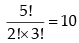Question 3: In how many different ways can four books A, B, C and D be arranged one above another in a vertical order such that the books A and B are never in continuous position?    
(a) 9
(b) 12
(c) 14
(d) 18

Let us take books A and B as one i.e., they are always continuous.
Now, number of books = 4 – 2 + 1 = 3
These three books can be arranged in 3! ways and also A and B can be arranged in 2 ways among themselves.
So, number of ways when books A and B are always continuous = 2 × 3!
Total number of ways of arrangement of A, B, C and D = 4!
Hence, number of ways when A and B are never continuous = Total number of ways – number of ways when A and B always continuous = 4! – 2 × 3! = 12

Question 4: Groups each containing 3 boys are to be formed out of 5 boys - A, B,C, D and E such that no one group contains both C and D together. What is the maximum number of such different groups?    
(a) 5
(b) 6
(c) 7
(d) 8

Total number of arrangements, when any 3 boys are selected out of 5 = 5C3. Now, when groups contains both C and D, then their selection is fixed and the remaining 1 boy can be selected out of the remaining 3 boys. It can be done in 3Cways.
So, number of groups, when none contains both C and D = total number of arrangements-number of arrangements when group contains both C and D
= 5C3C1 = 10 – 3 = 7

Question 5: In how many maximum different ways can 3 identical balls be placed in the 12 squares (each ball to be placed in the exact centre of the squares and only one ball is to be placed in one square) shown in the figure given below such that they do not lie along the same straight line?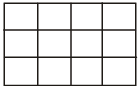(a) 144
(b) 200
(c) 204
(d) 216

3 balls can be placed be in any of the 12 squares in 12C3 ways.
Total number of arrangements = 12C3 = 220
Now, assume that balls lie along the some line.
There can be 3 cases:
Case I: When balls lie along the straight horizontal line. 3 balls can be put in any of the 4 boxes along the horizontal row in 4C3 ways.
Now, since there are 3 rows, so number of ways for case I = 4C3 × 3 = 12
Case II : When balls lie along the vertical straight line 3 balls can be put in any of the 3 boxes along the vertical row in 3C3 ways.
Now, as there are 4 vertical rows, so number of ways for Case II = 3C3 × 4 = 4
Case III : Balls lie along the 2 diagonal lines towards the left and 2 diagonal lines towards the right.
Number of ways = 2 + 2 = 4
Number of ways, when balls lie along the line = 12 + 4 + 4 = 20
Number of ways when balls don't lie along the line = Total number of ways – number of ways when balls lie along the line.  = 220 – 20 = 200.

Question 6: Three dice (each having six faces with each face having one number from 1 to 6) are rolled. What is the number of possible outcomes such that at least one dice shows the number 2?    
(a) 36
(b) 81
(c) 91
(d) 116

There can be 3 cases :

1. When one dice shows 2.
2. When two dice shows 2.
3. When three dices shows 2.

Case I : The dice which shows 2 can be selected out of the 3 dices in 3C1 ways.
Remaining 2 dices can have any 5 numbers except 2. So number of ways for them = 5C1 each, so no of ways when one dice shows 2 = 3C1 × 5C1 × 5C1.
Case II : Two dices, showing 2 can be selected out of the 3 dices in 3C2 ways and the rest one can have any 5 numbers except 2, so number of ways for the remaining 1 dice = 5.
So, number of ways, when two dices show 2
= 3C2 × 5
Case III : When three dices show 2 then these can be selected in 3C3 ways.
So, number of ways, when three dices show 2 = 3C3 = 1
As, either of these three cases are possible.
Hence, total number of ways = (3 × 5 × 5) + (3 × 5) + 1 = 91

Question 7: All the six letters of the name SACHIN are arranged to form different words without repeating any letter in any one word. The words so formed are then arranged as in a dictionary. What will be the position of the word SACHIN in that sequence?    
(a) 436
(b) 590
(c) 601
(d) 751

Out of the given letters in the word SACHIN, S is the last letter in the alphabetical order to start a word. If the word starts with A, then A can be kept fixed and the remaining letters can be arranged in 5! ways.
Similarly, number of words starting with C = 5!
Number of words starting with H = 5!
Number of words starting with I = 5!
Number of words starting with N = 5!
Now, when the word starts with S, then SACHIN is the first word in the alphabetical order to follow up.
So, Position of the word SACHIN = 5 (5!) + 1 = 601

Question 8: Five balls of different colours are to be placed in three different boxes such that any box contains at least one ball. What is the maximum number of different ways in which this can be done?    
(a) 90
(b) 120
(c) 150
(d) 180

These can be two cases :
Case I - One box contain 3 balls and rest two Contains 1 ball each.
Case II - One box contain 1 ball and rest two Contains 2 balls each.
Case 1 : Number of ways = 5C3 × 2C1 × 1C1 = 20
Now, these 3 boxes can be arranged in 3!/2! among themselves, as two of them contains similar number of balls.
So, number of ways = 20 x (3!/2!) = 60
Case II : Number of ways = 5C1 x 4C2 x 2C2 = 30
Also, these 3 boxes can be arranged among themselves in 3!/2!, as two of them contains similar number of balls.
Thus, number of ways = 30 x (3!/2!) = 90
Now, either of case (I) or case (II) is possible,
Hence, total number of ways = 60 + 90 = 150

Question 9: Amit has five friends: 3 girls and 2 boys. Amit’s wife also has 5 friends: 3 boys and 2 girls. In how many maximum number of different ways can they invite 2 boys and 2 girls such that two of them are Amit’s friends and two are his wife’s?    
(a) 24
(b) 38
(c) 46
(d) 58

There can be three cases :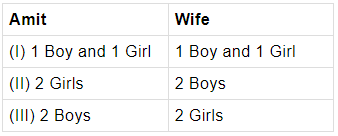Case I : number of ways = 2C1 × 3C1 × 3C1 × 2C1 = 36
Case II : number of ways = 3C2 × 3C2 = 9
Case III : number of ways = 2C2 × 2C2 = 1
Hence, total number of ways = 36 + 9 + 1 = 46

Question 10: In the figure shown below, what is the maximum number of different ways in which 8 identical balls can be placed in the small triangles 1, 2, 3 and 4 such that each triangle contains at least one ball?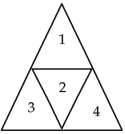(a) 32
(b) 35
(c) 44
(d) 56

There can be five cases :
Case I : First triangle can have 5 balls and rest three 1 each.
So, number of ways for (5, 1, 1, 1) = 4!/3!  = 4
(∵ Three triangles are having same number of balls)
Case II : Number of ways for (4, 2, 1, 1) = 4!/2! = 12

(∵ Two triangles are having same number of balls)
Case III : Similarly, number of ways for (2, 2, 2, 2) = 4!/4! = 1
Case IV : Number of ways for (3, 3, 1, 1)  =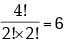Case V : Number of ways for (3, 2, 2, 1) = 4!/2! = 12
As, either of these five cases are possible,
Hence total number of ways  = 4 + 12 + 1 + 6 + 12 = 35

Question 11: Each of the 3 persons is to be given some identical items such that product of the numbers of items received by each of the three persons is equal to 30. In how many maximum different ways can this distribution be done?    
(a) 21
(b) 24
(c) 27
(d) 33

Suppose three people have been given a, b and c number of items.
Then, a × b × c = 30
Now, There can be 5 cases :
Case I : When one of them is given 30 items and rest two 1 item each.
So, number of ways for (30 × 1 × 1) = 3!/2! = 3
(As two of them have same number of items)
Case II : Similarly, number of ways for (10 × 3 × 1) = 3! = 6
Case III : Number of ways for (15 × 2 × 1) = 3! = 6
Case IV : Number of ways for (6 × 5 × 1) = 3! = 6
Case V : Number of ways for (5 × 3 × 2) = 3! = 6
Here, either of these 5 cases are possible.
Hence, total number of ways = 3 + 6 + 6 + 6 + 6 = 27

Question 12: Each of eight identical balls is to be placed in the squares shown in the figures given below in a horizontal direction such that one horizontal row contains six balls and the other horizontal row contains two balls. In how many maximum different ways can this be done?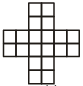(a) 38
(b) 28
(c) 16
(d) 14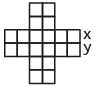There can be two cases:
Case (I) : When x row contains 6 balls:
Then the 2 balls can be arranged in y row in 6P2 ways = 15
or the 2 balls can be arranged in any of the 4 two box row in 4 ways.
So, no of ways, when x contains 6 balls = 15 + 4 = 19.
Case (II) : Similarly, no. of ways, when y row contains 6 balls = 19
As, either of case (I) or case (II) is possible,
Hence, total no. of ways = 19 + 19 = 38

Question 13: In a question paper, there are four multiple choice type questions. Each question has five choices with only one choice for its correct answer. What is the total number of ways in which a candidate will not get all the four answers correct?    
(a) 19
(b) 120
(c) 624
(d) 1024

Since, every question has five options, so no. of choices for each question = 5
∴  total no. of choices = 5 × 5 × 5 × 5 = 625
Now, no. of choices of all correct answer = 1
Hence, no of choices for all the four answers not correct
= total no. of choices – no. of choices of all correct answer = 625 – 1 = 624

Question 17: 3 digits are chosen at random from 1,2,3,4,5,6,7,8 and 9 without repeating any digit. What is the probability that their product is odd?    
(a) 2/3
(b) 5/108
(c) 5/42
(d) 8/42

Let E be the event of selecting the three numbers such that their product is odd and S be the sample space.
For the product to be odd, 3 numbers choosen must be odd.
∴ n (E) = 5C3
n (S) = 9C3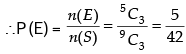Question 18: A mixed doubles tennis game is to be played between two teams (each team consists of one male and one female.) There are four married couples. No team is to consist of a husband and his wife. What is the maximum number of games that can be played?    
(a) 12
(b) 21
(c) 36
(d) 42

Married couples: MF  MF  MF  MF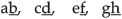Possible teams: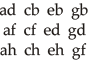Now, team ad can play only with: cb, cg, ch, eb, eh, gb, gf, i.e. 7
The same will apply with all teams.
So no. of total match = 12 × 7 = 84
Since every match includes 2 teams, so the No. of matches = 84/2 = 42

Question 19: There are three parallel straight lines. Two points, 'A' and 'B', are marked on the first line, points, 'C' and 'D' are marked on the second line; and points, 'E' and 'F', are marked on the third line. Each of these 6 points can move to any position on its respective straight line.
Consider the following statements:

1. The maximum number of triangles that can be drawn by joining these points is 18.
2. The minimum number of triangles that can be drawn by joining these points is zero.

Which of the statement(s) given above is/are correct?    [ 2006]
(a) 1 only
(b) 2 only
(c) Both 1 and 2
(d) Neither 1 nor 2

Maximum number of triangles can be formed by selecting 3, 4 or 5 points out of 6 at a time.
So, maximum no. of triangles = 6C3 + 6C4 + 6C5 which is clearly more than 18. Now, triangles formed will be minimum i.e., zero, when the points will overlap on the same line and all the points are along the same vertical line.

Question 20: In a tournament, each of the participants was to play one match against each of the other participants. Three players fell ill after each of them had played three matches and had to leave the tournament. What was the total number of participants at the beginning, if the total number of matches played was 75?    
(a) 08
(b) 10
(c) 12
(d) 15

Let the total no. of participants be 'n' at the beginning.
Players remaining after sometime = n – 3
Now, n–3C2 + (3 × 3) = 75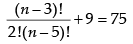n2 – 7n – 120 = 0
(n + 8) (n – 15) = 0
neglecting n = – 8, n = 15

Question 21: Each of two women and three men is to occupy one chair out of eight chairs, each of which numbered from 1 to 8. First, women are to occupy any two chairs from those numbered 1 to 4; and then the three men would occupy any, three chairs out of the remaining six chairs. What is the maximum number of different ways in which this can be done?    
(a) 40
(b) 132
(c) 1440
(d) 3660

2 Women can occupy 2 chairs out of the first four chairs in 4P2 ways. 3 men can be arranged in the remaining 6 chairs in 6P3 ways.
Hence, total no. of ways = 4P2 × 6P3 = 1440

Question 22: Ten identical particles are moving randomly inside a closed box. What is the probability that at any given point of time all the ten particles will be lying in the same half of the box?    
(a) 1/2
(b) 1/5
(c) 1/29
(d) 2/11

Probability of a particle lying in any particular half = 1/2
∴ Probability of all 10 particles lying in either 1st half or 2nd half =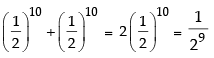Question 23: There are 10 identical coins and each one of them has ‘ H’ engraved on its one face and ‘T’ engraved on its other face. These 10 coins are lying on a table and each one of them has ‘H’ face as the upper face.
In one attempt, exactly four (neither more nor less) coins can be turned upside down. What is the minimum total number of attempts in which the ‘T’ faces of all the 10 coins can be brought to be the upper faces?    

(a) 4
(b) 7
(c) 8
(d) Not possible

On the first attempt four coins are overturned.
Now, six coins are left.
In the next turn, four more are overturned. Now only two would be left. We take one more from the left over two coins and any three from the previously turned ones. Finally, the leftover coin and the three coins from the presiding step which have already been turned twice can be overturned.
Thus, in four attempts, one can complete the process.

Question 24: A square is divided in to 9 identical smaller squares. Six identical balls are to be placed in these smaller square such that each of the three rows gets at least one ball (one ball in one square only). In how many different ways can this be done?     
(a) 27
(b) 36
(c) 54
(d) 81

Total number of ways in which 9 balls occupy any of the 6 squares = 9C6 = 84
Number of ways in which one row is not filled = 3
∴ Number of ways in which at least one ball occupies each row = 84 – 3 = 81

Question 25: 2 men and 1 women board a bus in which 5 seats are vacant. One of these five seats is reserved for ladies. A women may or may not sit on the seat reserved for ladies but a man can not sit on the seat reserved for ladies. In how many different ways can the five seats occupied by these passengers?    
(a) 15
(b) 36
(c) 48
(d) 60

There can be two cases :
(i)   Lady occupies the reserved seat
(ii)  Lady does not occupy the reserved seat.
(i)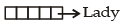Fixing one seat for the lady, 1st man can occupy any of there maining 4 seats in four ways and the 2nd man occupy any of the remaining 3 seats in three ways.
Hence, no of ways = 1 × 4 × 3 = 12
(ii)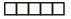Leaving the reserved seat, 1st man can occupy any of the4 seats in four ways. 2nd man can occupy any of the remaining 3 seats in three ways. Lady can occupy any of the remaining 2 seats in two ways.
Hence, no of ways = 4 × 3 × 2 = 24
Thus, Total no of ways = 12 + 24 = 36

Question 26: On a railway route between two places A and B, there are 20 stations on the way. If 4 new stations are to be added, how many types of new tickets will be required if each ticket is issued for a one way journey?    
(a) 14
(b) 48
(c) 96
(d) 108

For (10 + A + B) = 12 stations, no of tickets required, when 4 new stations are added for one way journey = 12 × 4 = 48
Also, each 4 new stations require (16 – 1) = 15 new tickets for one way journey.
∴ No. of tickets for 4 new stations = 15 × 4 = 60
Hence, total new tickets = 60 + 48 = 108

Question 27: Three students are picked at random from a school having a total of 1000 students. The probability that these three students will have identical date and month of their birth, is    
(a) 3/1000
(b) 3/365
(c) 1/3652
(d) None of these

For 1st student, Probability of selecting any one day as his birthday = 365/365 = 1
Now, the remaining two students to be selected must have same day as their birthday as for the 1st student.
Probability of rest two students, having the same birthday as that of the 1st student =  1/365 x 1/365
Hence, required probability =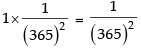Question 28: In how many different ways can six players be arranged in a line such that two of them, Ajit and Mukherjee, are never together?    
(a) 120
(b) 240
(c) 360
(d) 480

Total no of ways of arrangement for six players = 6!
Let us take Ajit and Mukerjee as one entity.
So now there are (6 – 2 + 1) = 5 players
These 5 players can be arranged in 5! ways and Ajit and Mukerjee can be arranged among themselves in 2! ways.
Thus, no of ways, when Ajit and Mukerjee are always together = 5 ! × 2!
Hence, no of ways when they are never together = Total no of ways – no of ways when they are always together
=   6 ! – (5 ! × 2 !) = 6 × 5 ! – (5 ! × 2 !) = 5 ! (6 – 2) = 480

Question 29: Nine different letters are to be dropped in three different letter boxes. In how many different ways can this be done?    
(a) 27
(b) 39
(c) 92
(d) 39 –3

First letter can be dropped into any of the 3 boxes. It can be done in 3 ways. Similarly second letter can also be dropped into any of the 3 boxes in 3 ways and so on. Hence, total no of ways = 3×3×3×.........upto 9 times = 39

Question 30: In a question of a test paper, there are five items each under List-A and List-B. The examinees are required to match each item under List-A with its corresponding correct item under List-B. Further, it is given that

1. no examinee has given the correct answer.
2. answers of no two examinees are identical

Which is the maximum number of examinees who took this test?     

(a) 24
(b) 26
(c) 119
(d) 129

Since, answers of no. two examinees are identical, so first item in List-A can be matched with any of the 5 items in List-B. It can be done in 5 ways. Similarly, 2nd item in List-A can be matched with any of the remaining 4 items in List-B. It can be done in 4 ways.
Continuing in the same way,
No of ways of arranging the items = 5 × 4 × 3 × 2 × 1=120
Now, in this arrangement there is one such arrangement, which is the correct answer.
∴ Maximum n umber of examinees = no. of ways of arrangement of items = 120 – 1 = 119.

Question 31: A two member committee comprising of one male and one female member is to be constituted out of five males and three females. Amongst the females, Mrs. A refused to be a member of the committee in which Mr. B is taken as the member. In how many different ways can the committee be constituted?    

(a) 11
(b) 12
(c) 13
(d) 14

For each combination, let us name the females and males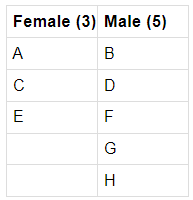Since A can’t go with B, it will make team with four males in four ways AD, AF, AG, AH. Since there is no restriction with female C and E, they may combine with 5 males in 5 different ways each.
Total number of ways = 4 + 5 + 5 = 14

Question 32: Three flags, each of different colour, are available for a military exercise. Using these flags, different codes can be generated by waving

(i) single flag of different colours or
(ii) any two flags in a different sequence of colour
OR
(iii) three flags in a different sequence of colours.

The maximum number of codes that can be generated, is     
(a) 6
(b) 9
(c) 15
(d) 18

(i) Number of ways of arranging three colours taken 1 at a time =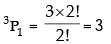(ii) Number of ways of arranging three colours taken 2 at a time =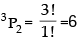(iii) Number of ways of arranging three colours taken 3 at a time = 3P3 = 6
Hence, Maximum no. of codes = No. of ways of arranging these flags = 3 + 6 + 6 = 15.

Question 33: A complete cycle of a traffic light takes 60 seconds. During each cycle the light is green for 25 seconds, yellow for 5 seconds and red for 30 seconds. At a randomly chosen time, the probability that the light will not be green, is    
(a) 1/3
(b) 1/4
(c) 5/12
(d) 7/12

Probability that the light is not green
= time for which light is not green/time taken for the entire cycle =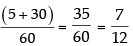Question 34: A bag contains 20 balls, 8 balls are green, 7 are white and 5 are red. What is minimum number of balls that must be picked up from the bag blind-folded (without replacing any of it) to be assured of picking atleast one ball of each colour?   
(a) 4
(b) 7
(c) 11
(d) 16

Since, 8 Green balls + 7 White balls = 15 balls
7 White balls + 5 Red balls = 12 balls
and 8 Green balls + 5 Red balls = 13 balls
Now, if we pick 15 balls, they may be white, green or red but if we pick 16 balls, then its certain that there will be atleast one ball of each colour.

Question 35: When three coins are tossed together, the probability that all coins have the same face up, is    
(a) 1/3
(b) 1/6
(c) 1/8
(d) 1/12

Probability of Head or Tail on the upper side for a coin = 1/2
∴ Probability of same side on the upper side for the three coins =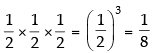The document Permutation, Combination, & Probability- Solved Questions(1995-2020)- 2 - Notes | Study UPSC Topic Wise Previous Year Questions - UPSC is a part of the UPSC Course UPSC Topic Wise Previous Year Questions.
All you need of UPSC at this link: UPSC

## UPSC Topic Wise Previous Year Questions

98 docs|91 tests
 Use Code STAYHOME200 and get INR 200 additional OFF

## UPSC Topic Wise Previous Year Questions

98 docs|91 tests

### How to Prepare for UPSC

Read our guide to prepare for UPSC which is created by Toppers & the best Teachers

Track your progress, build streaks, highlight & save important lessons and more!

,

,

,

,

,

,

,

,

,

,

,

,

,

,

,

,

,

,

,

,

,

,

,

,

,

,

,

;